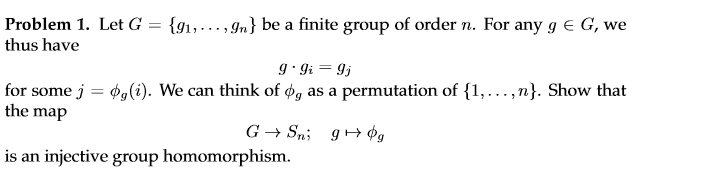# Abstract algebra, show that phi is a homomorphism

usn7564

## Homework Statement## The Attempt at a Solution

I'm very new to this kind of maths, so don't quite know how to get started. If I understood the question at all we have

$$g_i \mapsto \phi_i$$

and so I have a homomorphism if I can show that

$$\pi(g \cdot g_i) = \pi(g) \circ \pi(g_i)$$

I'm thinking it's trivially injective (might be way off here) because each g maps to a unique element in the symmetric group so there's not much to show.

But to show the homomorphism? Frankly don't have a clue
$$j = \phi_g (i)$$
$$\pi(g \cdot g_i) = \pi(g_j) = \phi_{g_j}$$

$$\pi(g) \circ \pi(g_i) = \phi_g \circ \phi_{g_i}$$

and then I come to a halt. How do I approach this?

Mandelbroth

## Homework Statement## The Attempt at a Solution

I'm very new to this kind of maths, so don't quite know how to get started. If I understood the question at all we have

$$g_i \mapsto \phi_i$$

and so I have a homomorphism if I can show that

$$\pi(g \cdot g_i) = \pi(g) \circ \pi(g_i)$$

I'm thinking it's trivially injective (might be way off here) because each g maps to a unique element in the symmetric group so there's not much to show.

But to show the homomorphism? Frankly don't have a clue
$$j = \phi_g (i)$$
$$\pi(g \cdot g_i) = \pi(g_j) = \phi_{g_j}$$

$$\pi(g) \circ \pi(g_i) = \phi_g \circ \phi_{g_i}$$

and then I come to a halt. How do I approach this?
It is trivially injective, so I'll let you show that in all of its glory if you really want to.

If it's alright with you, I'm going to drop the multiplication signs and just juxtapose two elements to multiply them.

We wish to show that ##\phi_{gg_i}=\phi_g\phi_{g_i}=\phi_{g_j}## for ##g,g_i,g_j\in G##. Do you see how to continue?

usn7564
It is trivially injective, so I'll let you show that in all of its glory if you really want to.

If it's alright with you, I'm going to drop the multiplication signs and just juxtapose two elements to multiply them.

We wish to show that ##\phi_{gg_i}=\phi_g\phi_{g_i}=\phi_{g_j}## for ##g,g_i,g_j\in G##. Do you see how to continue?
Not quite no, I mean I realize I have to find the connection between the expressions but don't know how to go about it. I'm assuming the j is a key here but as far as I can tell the permutation is more or less arbitrary so think I'm missing what information is entailed in the j.